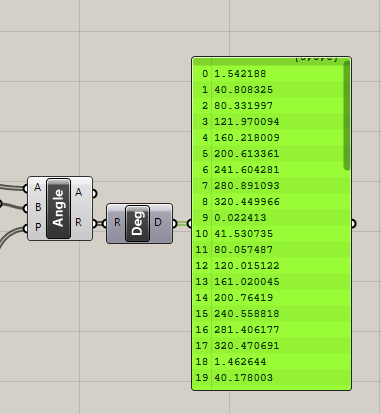# Continuously add rotational angle component?

I’m creating a spiral around cylinder. I’m looking for an easy way to continuously find the angle of a point. In simple terms, 2 cycles the angle would be 720 degrees. 2.5 cycles would be 900 degrees and so on.

My point are non uniform, my points could could be 358 degrees and my next point on the spiral could be 4 degrees. I could like this 4 degree values actually be 364.

Is there a setting in GH i’m missing? Or is this something I need to figure out in C# or Python?

Thanks!RelativeCoordinates360.gh (17.5 KB)

Does something like this work? (Assumes first vector as degree 0)
It gives the relative successive angles for a list, and you can use the Mass Addition component to get Partial Results that add up.

RelativeCoordinates360.gh (15.3 KB)

1 Like

HS_Kim,
I was unaware of the Point Cylinder component. This is a new tool I could use in the future.I’ll have to play around with it some more as I’ll eventually be working with no-cylinder models such as an extruded square.

you can also use twisted box and extract one curve